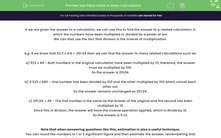# Use Place Value in Given Calculations

In this worksheet, students will use their knowledge of place value to find the answer to a calculation which is based on a related calculation which has the answer given. A calculator is not allowed throughout this activity.Key stage:  KS 4

Year:  GCSE

GCSE Subjects:   Maths

GCSE Boards:   OCR, AQA, Eduqas, Pearson Edexcel,

Curriculum topic:   Number Operations and Integers, Number

Curriculum subtopic:   Whole Number Theory Structure and Calculation

Difficulty level:#### Worksheet Overview

If we are given the answer to a calculation, we can use this to find the answer to a related calculation in which the numbers have been multiplied or divided by a power of ten.

We can also use the fact that division is the inverse of multiplication.

e.g. If we know that 52.3 x 4.8 = 251.04 then we can find the answer to many related calculations such as:

a) 523 x 48 - Both numbers in the original calculation have been multiplied by 10, therefore, the answer must be multiplied by 100.

b) 0.523 x 480 - One number has been divided by 100 and the other multiplied by 100 which cancel each other out.

So the answer remains unchanged as 251.04.

c) 251.04 ÷ 48 - The first number is the same as the answer of the original and the second has been multiplied by 10.

Since this is division, the answer will have the inverse operation applied, which is divide by 10.

Note that when answering questions like this, estimation is also a useful technique.

You can round the numbers to 1 or 2 significant figure and then estimate the answer, remembering that the digits will be the same as in the original calculation.

e.g. For c) above: 251.04 ÷ 48 ≈ 250 ÷ 50 = 5

So the answer must be 5.23 as it is close to our estimated guess of 5.

In this activity, you will use the information given in a calculation to enable you to work out related calculations by applying your knowledge of place value.

Good luck, place value superstar!

### What is EdPlace?

We're your National Curriculum aligned online education content provider helping each child succeed in English, maths and science from year 1 to GCSE. With an EdPlace account you’ll be able to track and measure progress, helping each child achieve their best. We build confidence and attainment by personalising each child’s learning at a level that suits them.

Get started